Math Concepts

# Complete Guide: How to subtract two numbers using Abacus?

2.3k views

 1 Introduction 2 What is an Abacus? 3 Basics of Abacus 4 Abacus Techniques 5 Solved Examples on Abacus Subtraction 6 Summary 7 FAQs 7 External References

12 November  2020

## Introduction

Can you imagine our world if numbers do not exist? How difficult would it be to count without numbers?

There was a time when written numbers did not exist. The earliest counting device would have been the human fingers or toes.

For greater or bigger numbers, people would depend upon natural resources available to them, such as pebbles, seashells, etc.

The abacus is the most ancient calculating device known. It has endured over time and is still in use.

The abacus was used as a counting tool before the advent of the Arabic numeral system.

Though the Abacus originated in Babylon it had traveled a long way from the Middle East to Japan and  China.

When it traveled from one country to another its translation changed as well. In China, it is called Suanpan. In Suanpan it has ⅖ decks.

The famous Japanese mathematician Seki Kawa replace ⅖ decks with ¼ decks and it is called Soroban. The modern-day abacus which we use today is the Soraban type of abacus.

Abacus Blogs
Abacus Anatomy & Construction Abacus History
Abacus Level I Abacus Level II
Abacus Counting Abacus Techniques
Abacus Multiplication Abacus Division
Negative Numbers in Abacus Abacus Basics

Here are some additional points that talk about How to subtract two numbers using Abacus?. To view them click on the Download button.

## What is an Abacus?

An abacus or a counting board consists of a wooden frame, rods, and beads.

Each rod represents a different place value—ones, tens, hundreds, thousands, and so on.

Each bead represents a number, usually 1 or 5, and can be moved along the rods.

Addition and subtraction can easily be performed by moving beads along the wires of the abacus. The beads that slide along a series of wires or rods set in a frame to represent the decimal places.

It can also be used to extract square-roots and cubic roots. The beads are manipulated with either the index finger or the thumb of one hand.

The abacus is typically constructed of various types of hardwoods and comes in varying sizes

The abacus frame has a series of vertical rods on which several wooden beads can slide freely. A horizontal beam separates the structure into two sections, known as the upper deck and the lower deck.

One could call it a precursor to the modern-day calculator. Merchants commonly used it in Europe and the Arabic world.

In the modern world of computers and calculators, it is used by traders, merchants, etc. in many parts of the world. It is still used to teach the basics of arithmetic to children.

## Basics of Abacus

We can use an abacus to solve all kinds of arithmetic operations such as addition, subtraction, multiplication, and division. It consists of rods and each rod contains some beads.

There are many kinds of abacus present but the Japanese abacus or soroban is the widely used one.

It has 4 beads at the bottom deck and 1 bead at the upper deck. Each rod can represent any number between 0 to 9 which is 10 numbers.

The beads above the horizontal crossbar are known as Heaven beads and the bottom one is known as earth beads.

We have to count or calculate by moving the beads up and down.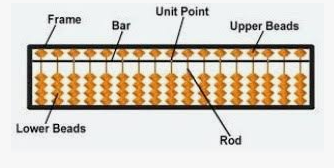Fig. Structure of Japanese abacus.

In Soroban, the beads are arranged according to place value from right to left.When no beads are touching the horizontal bar that means no number is showing. To show or count any number we have to move the beads.

Beads which are touching the bar represents a number according to their position.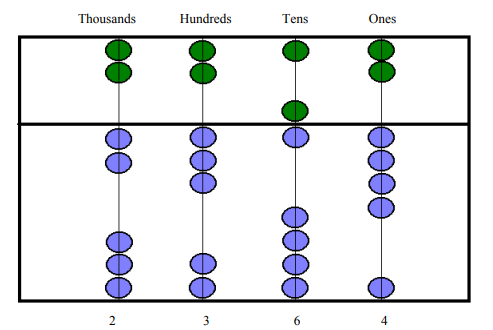The number showing in the above abacus is 2364 or two thousand three hundred sixty-four.

## Abacus techniques

### Counting

A. Arrange the Abacus: The beads of the abacus will be in its original position that means no beads will touch the reckoning bar. And showing the number zero.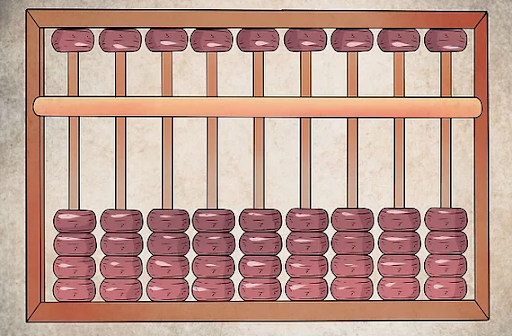B. Assign each vertical rod the place name: The rightmost rod named as Ones followed by tens, hundreds, and so on.

We can also assign a decimal place. If we want to represent a decimal number such as 10.5 then the rightmost column will be the tenth place, earlier it was one’s place and followed by the place of the whole numbers such as ones and tens.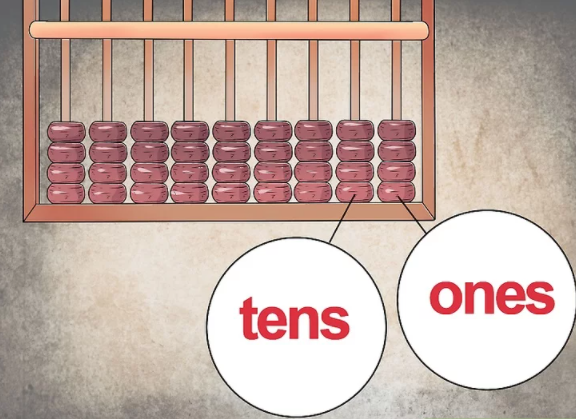C. We always have to start counting from the bottom deck. Number one should be represented by moving one bead of one’s place towards the horizontal reckoning bar.

Similarly, by moving the beads towards the reckoning the bar we can make any number.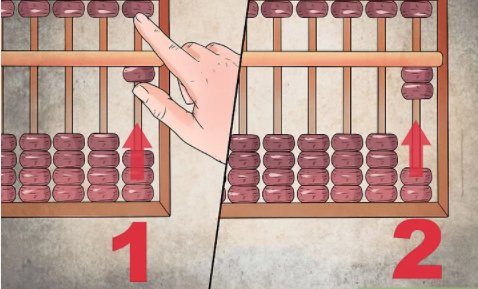As the bottom row has only four beads to make a number more than 4, we have to move back the bottom deck’s bead to its original position and bring down the heaven bead towards the reckoning bar.

To make 6 with the heaven beads, you need one earth bead touching the reckoning bar.

• To add a number, let’s take 1234 with another number, we need to make the number 1234 first by moving the beads according to their place.
• Now we have to start adding from the left that is the highest place, in this case, it is the thousand place, and have to add 1 and 5. And gradually to the lower places.
• Since the tens place has carried over(3+7=10) so have to add one bead at a hundred’s place and moving the tens’ bead to its original place making the value zero.  The same way has to add one’s place also.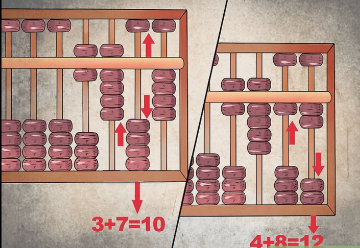Now have to read from the left side, 1234+5678= 6912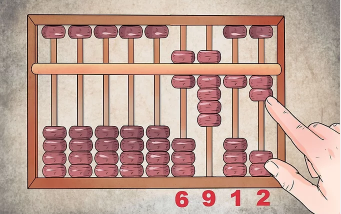• Subtraction is the reverse process of Addition. We have to make the greater number first by moving the beads in the same way. And have to borrow instead of carrying over.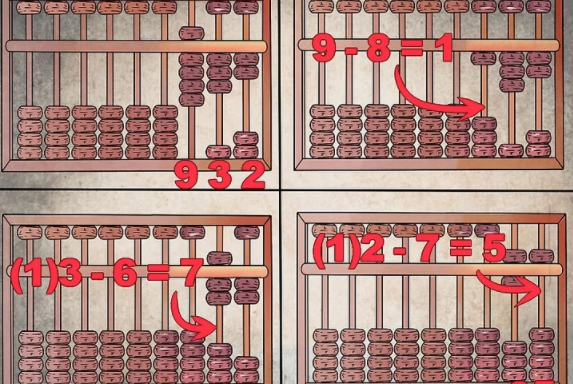The subtraction will be 932-867=65

## Solved Examples on Abacus Subtraction

It is better to understand subtraction using examples. Let us begin with something simple and try to calculate 102-78.

 Example 1

102-78=24

Step 1: Set 102 on rods ABC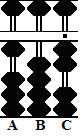Step 2: Subtract 7 from the tens rod B. Use the complement. Begin by subtracting 1 from hundreds rod A, then add the complementary 3 to rod B and so you are left with 32 on rods BC.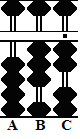Step 3:  Subtract 8 from units rod C. Use the complement again. Begin by subtracting 1 from hundreds rod A, then add the complementary 2 to rod C, leaving the answer 24 on rods BC.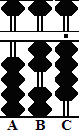Now, moving on to something that looks complicated but follows the same procedure for solving it.

 Example 2

146-57=89

Step 1: Set 146 on rods ABC.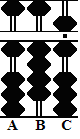Step 2: Subtract 5 from tens rod B. Use the complement. Begin by subtracting 1 from hundreds rod A, then add the complementary 5 to rod B, leaving 96 on rods ABC.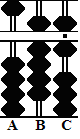Step 3: Subtract 7 from units rod C. Use the complement again. Begin by subtracting 1 from hundreds rod A, then add the complement 3 to rod C, leaving the answer 89 on rods ABC.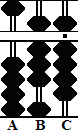Example 3

22.3-2.8=19.5

Step 1: Designate rod B as the unit rod. Set 22.3 on rods ABC.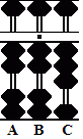Step 2: Subtract 2 from units rod B, leaving 20.3 on rods ABC.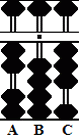Step 3: Subtract 8 from tenths rod C. Use the complement. Begin by subtracting 1 from units rod on B but here seems to be a problem. Rod B is empty. There's nothing there to subtract, so you have to go all the way over to rod A for help.

Step 4: Subtract 1 from tens rod A. Add 9 to rod B, then add the complementary 2 to rod C, leaving 19.5 on rods ABC.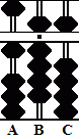Though it might look as though the last example is a bit of stretch, but with practice, this can seem pretty easy.

If you want to try something complicated, you can start solving examples such as 78-102 or 2.8-22.3.

Basically, you need to start addressing negative numbers on an abacus.

## Summary

The Abacus is the oldest counting equipment. From  5000 years ago to this 21st century, the abacus went through many transitions.

It started with a tray of sand and became a computerized calculating device. It is a huge journey but the sole purpose of the abacus remains the same, making the calculation easier.

Though Abacus is now replaced by electronic calculators and computers, as a mathematical teaching tool, its role is still intact.

Abacus is very useful and important for blind people, which is known as Cranmer Abacus.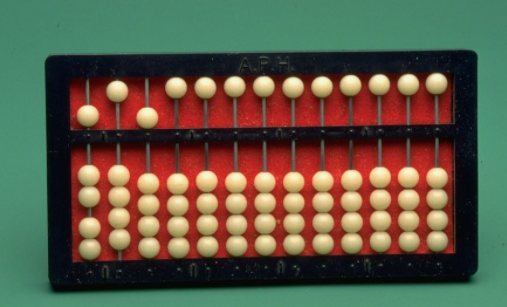Now that you know how to do subtraction using abacus, you can try more subtraction problems to strengthen your understanding.

## Related Blogs

Abacus Blogs
Abacus Anatomy & Construction Abacus History
Abacus Level I Abacus Level II
Abacus Counting Abacus Techniques
Abacus Multiplication Abacus Division
Negative Numbers in Abacus Abacus Basics

Cuemath, student-friendly mathematics and coding platform, conducts regular Online Live Classes for academics and skill-development, and their Mental Math App, on both iOS and Android, is a one-stop solution for kids to develop multiple skills.

Check out the fee structure for all grades and book a trial class today!

## What is an Abacus?

An Abacus is a manual aid for calculating which consists of beads that can be moved up and down on a series of sticks or strings within a usually wooden frame. The Abacus itself doesn't calculate; it's merely a device for helping a human being calculate by remembering what has been counted.

## Where was the Abacus invented?

The type of Abacus most commonly used today was invented in China around the 2nd century B.C. However, Abacus-like devices are first attested from ancient Mesopotamia around 2700 B.C.!

## Where was Abacus first used?

The Abacus (plural abaci or abacuses), also called a counting frame, is a calculating tool used in the ancient Near East, Europe, China, and Russia, centuries before the adoption of the written Arabic numeral system. The exact origin of the Abacus is still unknown.

## What are the advantages of learning how to use an Abacus?

Mathematical skills lay a secure foundation for higher classes.
Abacus education improves the skills of

• Visualization (photographic memory)
• Concentration
• Listening Skills
• Memory, Speed
• Accuracy
• Creativity
• Self Confidence
• Self-Reliance resulting in Whole Brain Development

## Is it good for children to use an abacus?

Yes, an abacus is an excellent tool for teaching children basic math. The different senses involved in using an abacus, like sight and touch, can also reinforce the lessons

## External References

Related Articles
Award-winning math curriculum, FREE for a year